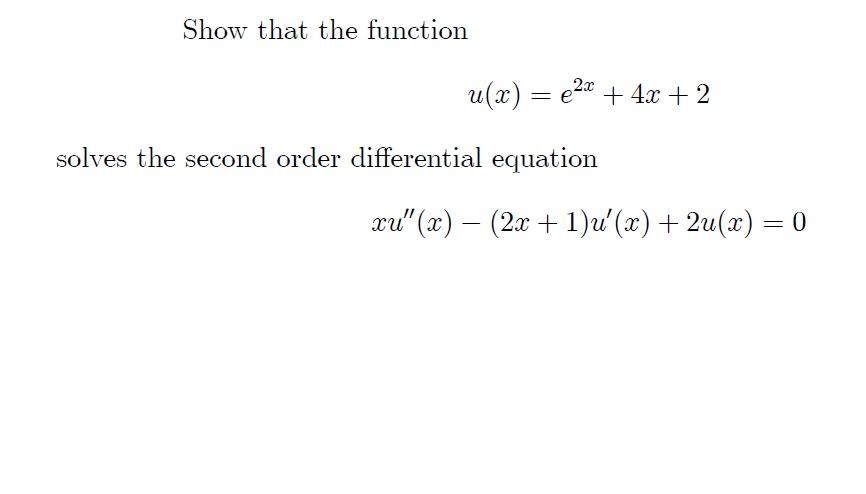### Create an Account

Already have account?

### Forgot Your Password ?

Home / Questions / Show that the function u(x) = €2x + 4x + 2 solves the second order differential equation x...

# Show that the function u(x) = €2x + 4x + 2 solves the second order differential equation xu" (x) – (2x + 1)u'(x) + 2u(x) = 0

Show that the function u(x) = €2x + 4x + 2 solves the second order differential equation xu" (x) – (2x + 1)u'(x) + 2u(x) = 0Apr 14 2021 View more View Less

#### Answer (Solved)Subscribe To Get Solution